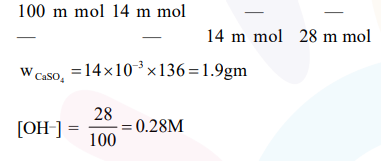# A mixture of 100 m mol of Ca(OH)2 and 2g of

Question:

A mixture of $100 \mathrm{~m} \mathrm{~mol}$ of $\mathrm{Ca}(\mathrm{OH})_{2}$ and $2 \mathrm{~g}$ of sodium sulphate was dissolved in water and the volume was made up to $100 \mathrm{~mL}$. The mass of calcium sulphate formed and the concentration of $\mathrm{OH}^{-}$in resulting solution, respectively, are : (Molar mass of $\mathrm{Ca}(\mathrm{OH})_{2}, \mathrm{Na}_{2} \mathrm{SO}_{4}$ and $\mathrm{CaSO}_{4}$ are 74,143 and $136 \mathrm{~g} \mathrm{~mol}^{-1}$, respectively; $\mathrm{K}_{\text {sp }}$ of $\mathrm{Ca}(\mathrm{OH})_{2}$ is $\left.5.5 \times 10^{-6}\right)$

1. $1.9 \mathrm{~g}, 0.14 \mathrm{~mol} \mathrm{~L}^{-1}$

2. $13.6 \mathrm{~g}, 0.14 \mathrm{~mol} \mathrm{~L}^{-1}$

3. $1.9 \mathrm{~g}, 0.28 \mathrm{~mol} \mathrm{~L}-1$

4. $13.6 \mathrm{~g}, 0.28 \mathrm{~mol} \mathrm{~L}^{-1}$

Correct Option: , 3

Solution:

$\mathrm{Ca}(\mathrm{OH})_{2}+\mathrm{Na}_{2} \mathrm{SO}_{4} \longrightarrow \mathrm{CaSO}_{4}+2 \mathrm{NaOH}$